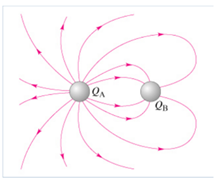Problem: The electric field lines are shown for a system of two point charges. Which of the following could represent the magnitudes and signs of QA and QB? In the following, take q to be a positive quantity. a) QA = +q, QB = −q b) QA = +7q, QB = −3q c) QA = +3q, QB = −7q d) QA = −3q, QB = +7q B e) QA = −7q, QB = +3q

87% (30 ratings)
Problem Details

The electric field lines are shown for a system of two point charges. Which of the following could represent the magnitudes and signs of QA and QB? In the following, take q to be a positive quantity.

a) QA = +q, QB = −q

b) QA = +7q, QB = −3q

c) QA = +3q, QB = −7q

d) QA = −3q, QB = +7q B

e) QA = −7q, QB = +3q